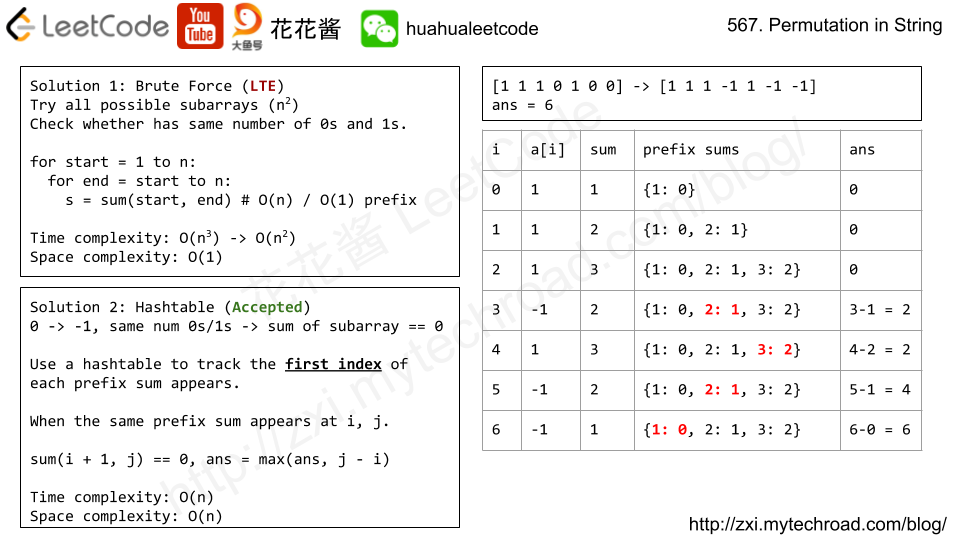# Problem

A sequence X_1, X_2, ..., X_n is fibonacci-like if:

• n >= 3
• X_i + X_{i+1} = X_{i+2} for all i + 2 <= n

Given a strictly increasing array A of positive integers forming a sequence, find the length of the longest fibonacci-like subsequence of A.  If one does not exist, return 0.

(Recall that a subsequence is derived from another sequence A by deleting any number of elements (including none) from A, without changing the order of the remaining elements.  For example, [3, 5, 8] is a subsequence of [3, 4, 5, 6, 7, 8].)

Example 1:

Input: [1,2,3,4,5,6,7,8]
Output: 5
Explanation:
The longest subsequence that is fibonacci-like: [1,2,3,5,8].


Example 2:

Input: [1,3,7,11,12,14,18]
Output: 3
Explanation:
The longest subsequence that is fibonacci-like:
[1,11,12], [3,11,14] or [7,11,18].


Note:

• 3 <= A.length <= 1000
• 1 <= A < A < ... < A[A.length - 1] <= 10^9
• (The time limit has been reduced by 50% for submissions in Java, C, and C++.)

# Solution 2: HashTable

Time complexity: O(n^3)

Space complexity: O(n)

# Problem

Starting with a positive integer N, we reorder the digits in any order (including the original order) such that the leading digit is not zero.

Return true if and only if we can do this in a way such that the resulting number is a power of 2.

Example 1:

Input: 1
Output: true


Example 2:

Input: 10
Output: false


Example 3:

Input: 16
Output: true


Example 4:

Input: 24
Output: false


Example 5:

Note:

1. 1 <= N <= 10^9

# Solution: HashTable

Compare the counter of digit string with that of all power of 2s.

e.g. 64 -> {4: 1, 6: 1} == 46 {4:1, 6: 1}

Time complexity: O(1)

Space complexity: O(1)

C++

# Problem

Given two arrays, write a function to compute their intersection.

Example:
Given nums1 = [1, 2, 2, 1]nums2 = [2, 2], return [2, 2].

Note:

• Each element in the result should appear as many times as it shows in both arrays.
• The result can be in any order.

• What if the given array is already sorted? How would you optimize your algorithm?
• What if nums1‘s size is small compared to nums2‘s size? Which algorithm is better?
• What if elements of nums2 are stored on disk, and the memory is limited such that you cannot load all elements into the memory at once?

# Solution1: Hashtable

Time complexity: O(m + n)

Space complexity: O(m)

# Problem

Given a list of non-negative numbers and a target integer k, write a function to check if the array has a continuous subarray of size at least 2 that sums up to the multiple of k, that is, sums up to n*k where n is also an integer.

Example 1:

Input: [23, 2, 4, 6, 7],  k=6
Output: True
Explanation: Because [2, 4] is a continuous subarray of size 2 and sums up to 6.


Example 2:

Input: [23, 2, 6, 4, 7],  k=6
Output: True
Explanation: Because [23, 2, 6, 4, 7] is an continuous subarray of size 5 and sums up to 42.


Note:

1. The length of the array won’t exceed 10,000.
2. You may assume the sum of all the numbers is in the range of a signed 32-bit integer.

# Special case:

nums = [0,0], k = 0, return = True

# Solution: Prefix Sum Reminder

Time complexity: O(n)

Space complexity: O(min(n, k))

# Problem

Given a binary array, find the maximum length of a contiguous subarray with equal number of 0 and 1.

Example 1:

Input: [0,1]
Output: 2
Explanation: [0, 1] is the longest contiguous subarray with equal number of 0 and 1.


Example 2:

Input: [0,1,0]
Output: 2
Explanation: [0, 1] (or [1, 0]) is a longest contiguous subarray with equal number of 0 and 1.


Note: The length of the given binary array will not exceed 50,000.# Solution: HashTable

Prefix sum + hashtable

Time complexity: O(n)

Space complexity: O(n)

V2: Using array instead of a hashtable

Time complexity: O(2*n + 1 + n)

Space complexity: O(2*n + 1)

Mission News Theme by Compete Themes.